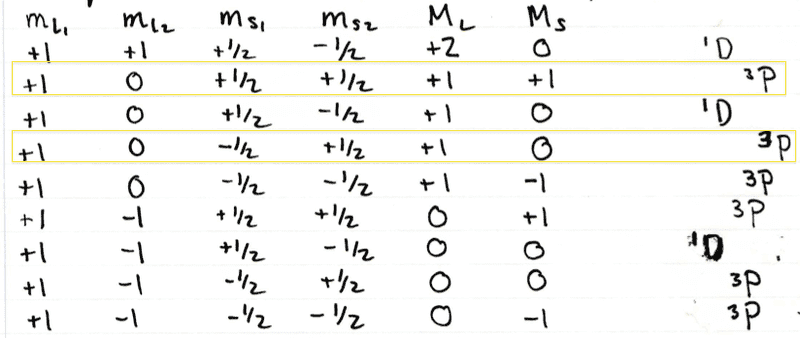# LS coupling for identical electrons

mk_gm1
Let's say I'm considering the $$3p^2$$ electrons. From the Pauli Exclusion Principle, we know that two electrons cannot have the same state, which in this case means ml and ms cannot both be the same for each electron.

What this means is that the following 6 terms must not be allowed:

$$m_{l1} \hspace{0.1 in} m_{l2} \hspace{0.1 in} m_{s1} \hspace{0.1 in} m_{s2}$$

$$-1 \hspace{0.1 in} -1 \hspace{0.1 in} \downarrow \hspace{0.3 in} \downarrow$$
$$-1 \hspace{0.1 in} -1 \hspace{0.1 in} \uparrow \hspace{0.3 in} \uparrow$$
$$0 \hspace{0.4 in} 0 \hspace{0.3 in} \downarrow \hspace{0.3 in} \downarrow$$
$$0 \hspace{0.4 in} 0 \hspace{0.3 in} \uparrow \hspace{0.3 in} \uparrow$$
$$+1 \hspace{0.1 in} +1 \hspace{0.1 in} \downarrow \hspace{0.3 in} \downarrow$$
$$+1 \hspace{0.1 in} +1 \hspace{0.1 in} \uparrow \hspace{0.3 in} \uparrow$$

These correspond to $$M_L=\sum m_{li} = -2, -2, 0, 0, 2, 2$$ and $$M_S = \sum m_{si} = -1, 1, -1, 1, -1, 1$$ respectively.

My question is this - how does this lead to the conclusion that the allowed terms are 1S, 1D and 3P ? For example, there's a ML = 0, MS= -1 term in both 3S and 3P - why do we disallow one and not the other?

Also, what leads us to disallow 1P (for which ML=-1, 0, 1 and MS=0)? Surely the only way to have MS = 0 is to have $$\downarrow_1 \hspace{0.1 in} \uparrow_2$$ or vice versa, and hence $$m_{s1} \neq m_{s2}$$ and we have no violation of the Pauli Exclusion Principle?

## Answers and Replies

ManyNames
What do you mean by ''why do we disallow one and not the other?''

mk_gm1
Ok let me try and clarify what I'm confused by. Have a look at the following table which I have taken from my notes:In this table we are looking at part of the identical (np)2 configuration. There are 15 possible configurations, all of which I haven't posted.

Take a look at the 2nd row. I can see why this state corresponds to the 3P term. For it to be a P term, it must have L = 1 where $$L \geq |M_L|$$. It clearly has this. For it to be a 3P term, the multiplicity 2S+1=3 i.e. S=1 where $$S \geq | M_S |$$. This is obvious.

Now if you look at the 4th row, I am not sure why it also has to be a 3P state . I understand that it can be (i.e. that the 3P term includes this state), but this could equally well be a 1P state, no? This is because here S=0. Therefore why do we choose one and not the other?